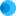Intuit HelpIntuit

# Calculating income into US dollars on Form 5471, Schedule C Income Statement in ProConnect

SOLVEDby IntuitUpdated June 27, 2023

This article explains what to do if a translation to US dollars isn't calculating on Form 5471, on page 2 of Schedule C Income Statement.

• The amounts entered for Form 5471, Schedule C can be either in functional currency amounts or US dollar amounts.
• If no entry is made in the Translation Rate input field under the Schedule C section, ProConnect will assume the amounts are US dollar amounts and won't complete the Functional Currency column.

## To generate a Schedule C Income Statement:

1. From the Input Return tab, go to Miscellaneous Forms  Info Return of US Persons (5471).
2. Verify the Category 3 filer or Category 4 filer boxes are checked.
3. Go to the Sch. C & F tab.
4. Locate the Translation Rates subsection.
5. Enter the Average or Year end rates (if applicable).
6. Scroll down to the Schedule C- Income Statement section.
7. Enter a '1', '2', or rate in, Translation (1=average rate, 2=year end, or rate (xxx.xxxxxxx)) if no entry, U.S. dollars will be assumed to be entered).
• If a 1 is entered, ProConnect will use the entry made in Average (Step 5) to calculate the US Dollars column.
• If a 2 is entered, ProConnect will use the entry made in Year End (Step 5) to calculate the US Dollars column.
• If a rate (xxx.xxxxxxx) is entered, the amounts will print in the Functional Currency column and be divided by the Translation input field amount (xxx.xxxxxxx) to complete the US Dollars column.

If you don't enter the corresponding rate in the appropriate fields, the translation calculation can't be done and no amounts will appear in the US Dollars column.